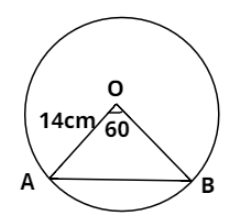Courses
Courses for Kids
Free study material
Free LIVE classes
MoreLIVE
Join Vedantu’s FREE Mastercalss

# A chord AB of a circle, of radius 14 cm makes an angle of ${60^ \circ }$at the center of the circle. Find the area of the minor segment of the circle.Verified
361.2k+ views
Hint: Analyze the situation with a diagram. Calculate the area of the minor sector of the circle first and then subtract the area of the triangle formed by the chord joining with the center from it.

According to the information given in the question, consider the above figure. We have to calculate the area of the minor segment of the circle divided by chord AB. Clearly, the minor segment of the circle is lying at the bottom of chord AB.
AB is subtending angle ${60^ \circ }$ at the center and the radius of the circle is 14 cm.
Area of circle $= \pi \times {\left( {14} \right)^2} = 196\pi$
Area of sector OAB of circle $= \dfrac{{{{60}^ \circ }}}{{{{360}^ \circ }}} \times 196\pi = \dfrac{1}{6} \times 196\pi$
Now, $\Delta OAB$ is an isosceles triangle because OA and OB are the radius of the circle. We know that:
Area of triangle $= \dfrac{1}{2}ab\sin \theta$, applying this formula $\Delta OAB$, we’ll get:
$\Rightarrow$Area ($\Delta OAB$) $= \dfrac{1}{2} \times OA \times OB \times \sin {60^ \circ } = \dfrac{1}{2} \times 14 \times 14 \times \dfrac{{\sqrt 3 }}{2}$,
$\Rightarrow$Area ($\Delta OAB$) $= 49\sqrt 3$
Now the area of minor segment is equal to the difference between the area of sector OAB and area of triangle AOB:
$\Rightarrow$Area of minor segment $= \dfrac{1}{6} \times 196\pi - 49\sqrt 3 = \dfrac{{196 \times 22}}{{6 \times 7}} - 84.87$,
$\Rightarrow$Area of minor segment $= 102.67 - 84.87 = 17.8$
Thus the area of the minor segment is 17.8 square cm.

Note: If $\theta$is the angle subtended by a sector of a circle of radius r at the center, then the area of the sector is given as:
$\Rightarrow$Area of sector $= \dfrac{\theta }{{{{360}^ \circ }}} \times \pi {r^2}$Last updated date: 18th Sep 2023
Total views: 361.2k
Views today: 4.61k